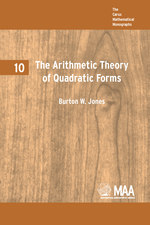# The Arithmetic Theory of Quadratic Forms

BURTON W. JONES
Volume: 10
Edition: 1
Pages: 223
https://www.jstor.org/stable/10.4169/j.ctt5hh98f

1. Front Matter
(pp. i-vi)
2. INTRODUCTION
(pp. vii-viii)
Burton W. Jones

The arithmetic theory of quadratic forms may be said to have begun with Fermat in 1654 who showed, among other things, that every prime of the form$8n + 1$is representable in the form${x^2} + 2{y^2}$forxandyintegers. Gauss was the first systematically to deal with quadratic forms and from that time, names associated with quadratic forms were most of the names in mathematics, with Dirichlet playing a leading role. H. J. S. Smith, in the latter part of the nineteenth century and Minkowski, in the first part of this, made notable and systematic contributions to the theory. In...

(pp. ix-x)
4. Chapter I FORMS WITH REAL COEFFICIENTS
(pp. 1-16)

Though in this chapter we shall be dealing primarily with quadratic forms with real coefficients, most of the definitions carry over into later theory and, by way of introduction, the questions we shall ask and answer will, for the most part, have their analogues later in the book.

Of fundamental importance is the notion of congruence which appears not only in this chapter but in our treatment of forms with rational andp-adic coefficients and which is closely allied to the notion of equivalence. Let

$f = \sum\limits_{i,j = 1}^n {{a_{ij}}} {x_i}{x_j}$and$g = \sum\limits_{i,j = 1}^n {{b_{ij}}} {y_i}{y_j}$

be two quadratic forms with real coefficients and${a_{ij}} = {a_{ji}}$,${b_{ij}} = {b_{ji}}$for all...

5. Chapter II FORMS WITH p-ADIC COEFFICIENTS
(pp. 17-55)

It turns out to be the case that the criteria for congruence and representation of numbers by forms with rational coefficients can be reduced to consideration of like problems for forms withp-adic coefficients. As was stated in the introduction, the results of this chapter could be stated largely as Minkowski gave them in terms of congruences. Pall has done just this. But since the gain in their introduction seems to justify such treatment, we prove our results in terms ofp-adic numbers.

Forpa prime number, we define ap-adic numberto be any formal series

(3)$\alpha = {\alpha _{ - r}}{p^{ - r}} + {\alpha _{ - r + 1}}{p^{ - r + 1}} + ... + {\alpha _{ - 1}}{p^{ - 1}} + {\alpha _0} + {\alpha _1}p + {\alpha _2}{p^2} + ...$...

6. Chapter III FORMS WITH RATIONAL COEFFICIENTS
(pp. 56-81)

When two forms with rational coefficients may be taken into each other by linear transformations with rational elements, the forms are frequently called rationally equivalent. But, to be consistent with our terminology, we shall call two such formsrationally congruentorcongruentin the field of rational numbers and reserve the term “equivalent” for transformations with coefficients in a ring. We shall see that there is an intimate connection between the fundamental results of this chapter and those of the previous chapter.

Since the rational numbers form a field we have shown in theorem 1 that every form is rationally...

7. Chapter IV FORMS WITH COEFFICIENTS IN $R(p)$
(pp. 82-104)

We further restrict our forms in this chapter by requiring that their coefficients bep-adic integers, that is, in$R(p)$. As the theory for$F(p)$led to results for forms with rational coefficients, so the theory for$R(p)$leads to results for forms with integer coefficients though, as we shall see, there is here an intermediate case which has no previous analogy. Suppose a transformationTwith elements in$R(p)$takes a formfinto a formgwhere both forms are in$R(p)$and have non-zero determinants${d_f}$and${d_g}$, respectively, and a transformationSin$R(p)$takesg...

8. Chapter V GENERA AND SEMI-EQUIVALENCE
(pp. 105-121)

Reasoning by analogy from the results of chapter III one might deduce that the function of this chapter would be to prove that if a formfwith integral coefficients represents a numberNin$R(p)$for allpand in the ield of reals, then there would be integer values of the variables offfor whichf=N. One might also suppose that a similar result would hold for equivalence in$R(p)$and in the ring of integers. But, while it is true that equivalence (or representation) in the ring of integers implies equivalence in$R(p)$for...

9. Chapter VI REPRESENTATIONS BY FORMS
(pp. 122-138)

Iffandgare forms with integer coefficients andnandmvariables respectively (n>m) the results of the last chapter give us methods of finding whether or not some form in the genus offrepresentsgintegrally. Corollary 44a or 44b may be used to show that the existence of such a representation depends on the solvability of the congruence$f \equiv g(\bmod 8|g|P)$wherePis the product of the odd primes in$\left| g \right| \cdot \left| f \right|$, or on the existence of representations in$R(p)$for allpdividing$2\left| f \right|\left| g \right|$. When there is only one class in the genus of...

10. Chapter VII BINARY FORMS
(pp. 139-185)

Most of the results in this chapter are classical—some dating back to the time of Gauss and earlier—and can be derived independently of the previous general theory. But viewing the binary forms as special cases of our previous results illuminates the general theory on the one hand and economizes labor on the other. Furthermore certain problems, such as the determination of all automorphs, inaccessible in the general case, can be completely solved for binary forms.

Since much of the theory of binary forms was developed in advance of the general theory there is a wide divergence in the...

11. Chapter VIII TERNARY QUADRATIC FORMS
(pp. 186-196)

In this chapter we shall consider only forms whose matrices have integral elements. We have seen in theorem 40 that the genus of a form is characterized by the forms to which it is congruent for an arbitrary modulus. In the previous chapter we showed that the genus of a primitive binary form could be characterized by the quadratic character of numbers represented by the form, where the characters were taken with respect to the prime factors of twice the determinant. For ternary forms (but not for forms in more than three variables) the genus may also be characterized by...

12. BIBLIOGRAPHY
(pp. 196-197)
13. PROBLEMS
(pp. 198-208)
14. THEOREM INDEX
(pp. 209-210)
15. INDEX
(pp. 211-212)